# 5 Bubble Chart

This chapter describes the bubble chart, a two-axis chart that plots bubbles for the data points in a series.

Each data item can be defined by two or three parameters. Figure 5-1 shows a typical bubble chart, where each data item is presented by the three following parameters: X value, Y value, and the radius of the bubble.

Figure 5-1 Typical Bubble Chart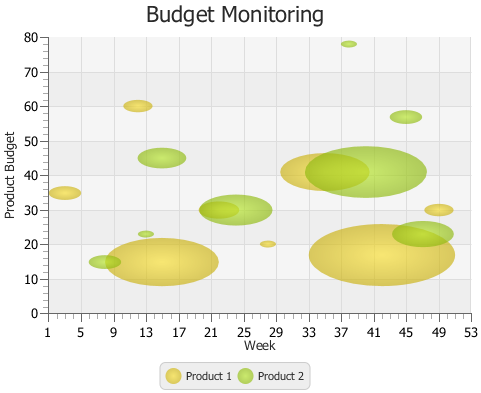Description of "Figure 5-1 Typical Bubble Chart"

## Creating a Bubble Chart

To create a bubble chart in your JavaFX application, at minimum, you must instantiate the `BubbleChart` class, define horizontal and vertical axes, and specify one or more series of data by using constructors of the `XYChart.Data` class with two or three parameters. Example 5-1 creates a bubble chart with two series of data. Each data item is represented by X and Y values: a number of a week and an amount of product budget.

Example 5-1 Creating a Bubble Chart with Two Data Parameters

```import javafx.application.Application;
import javafx.scene.Scene;
import javafx.scene.chart.BubbleChart;
import javafx.scene.chart.NumberAxis;
import javafx.scene.chart.XYChart;
import javafx.stage.Stage;

public class BubbleChartSample extends Application {

@Override public void start(Stage stage) {
stage.setTitle("Bubble Chart Sample");
final NumberAxis xAxis = new NumberAxis(1, 53, 4);
final NumberAxis yAxis = new NumberAxis(0, 80, 10);
final BubbleChart<Number,Number> blc = new
BubbleChart<Number,Number>(xAxis,yAxis);
xAxis.setLabel("Week");
yAxis.setLabel("Product Budget");
blc.setTitle("Budget Monitoring");

XYChart.Series series1 = new XYChart.Series();
series1.setName("Product 1");
series1.getData().add(new XYChart.Data(3, 35));
series1.getData().add(new XYChart.Data(12, 60));
series1.getData().add(new XYChart.Data(15, 15));
series1.getData().add(new XYChart.Data(22, 30));
series1.getData().add(new XYChart.Data(28, 20));
series1.getData().add(new XYChart.Data(35, 41));
series1.getData().add(new XYChart.Data(42, 17));
series1.getData().add(new XYChart.Data(49, 30));

XYChart.Series series2 = new XYChart.Series();
series2.setName("Product 2");
series2.getData().add(new XYChart.Data(8, 15));
series2.getData().add(new XYChart.Data(13, 23));
series2.getData().add(new XYChart.Data(15, 45));
series2.getData().add(new XYChart.Data(24, 30));
series2.getData().add(new XYChart.Data(38, 78));
series2.getData().add(new XYChart.Data(40, 41));
series2.getData().add(new XYChart.Data(45, 57));
series2.getData().add(new XYChart.Data(47, 23));

Scene scene  = new Scene(blc);
blc.getData().addAll(series1, series2);
stage.setScene(scene);
stage.show();
}

public static void main(String[] args) {
launch(args);
}
}
```

The result of compiling and running this application is shown in Figure 5-2.

Figure 5-2 Bubble Chart with Two Data Parameters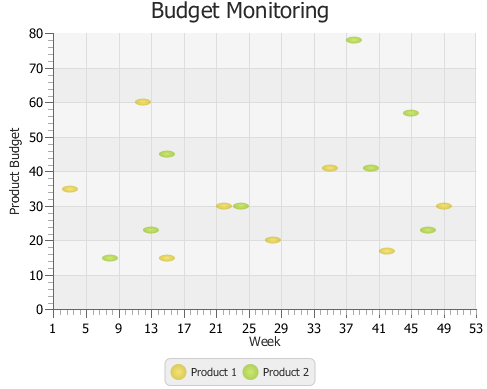Description of "Figure 5-2 Bubble Chart with Two Data Parameters"

This application defines lower and upper boundaries of the data ranges and the tick units within the constructors of the `NumberAxis` class. Additionally, the minor tick count is set to 4, so that each minor tick corresponds to a particular week.

To indicate that the vertical axis renders the amount of money in US dollars, use a tick label formatter as shown in Example 5-2.

Example 5-2 Formatting Tick Labels

```yAxis.setTickLabelFormatter(new NumberAxis.DefaultFormatter(yAxis,"\$ ",null));
```

The `NumberAxis.DefaultFormatter` class adds prefixes and suffixes to the tick labels of the specified axis. In Example 5-2, the formatter defines a dollar sign (\$) prefix for each tick label of the vertical axis. The `null` value for the suffix parameter indicates that no suffixes are added. Figure 5-3 shows the bubble chart after the formatting has been applied.

Figure 5-3 Specifying Prefixes for Tick Labels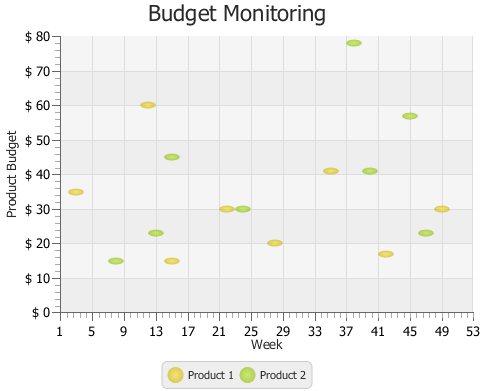Description of "Figure 5-3 Specifying Prefixes for Tick Labels"

## Using the Extra Value Property

The bubble chart shown in Figure 5-1 provides information about budgets of two products for the period of a year. However, you can enhance this application and benefit from the additional capabilities of the `BubbleChart` class. Use the `extraValue` property and define three parameters in `XYChart.Data` objects when specifying the series of data for your bubble chart.

The code fragment shown in Example 5-3 demonstrates how to modify data series for the Budget Monitoring application, so that each bubble shows the percentage of consumed budget for a particular product. The third parameter in the `XYChart.Data` object defines the radius of each bubble: the bigger the radius, the higher the percentage of the budget consumed. Thus, a radius of 7.5 corresponds to consuming 75% of the budget, 5.5 to 55%, and so on.

Example 5-3 Adding Extra Value

```XYChart.Series series1 = new XYChart.Series();
series1.setName("Product 1");
series1.getData().add(new XYChart.Data(3, 35, 2));
series1.getData().add(new XYChart.Data(12, 60, 1.8));
series1.getData().add(new XYChart.Data(15, 15, 7));
series1.getData().add(new XYChart.Data(22, 30, 2.5));
series1.getData().add(new XYChart.Data(28, 20, 1));
series1.getData().add(new XYChart.Data(35, 41, 5.5));
series1.getData().add(new XYChart.Data(42, 17, 9));
series1.getData().add(new XYChart.Data(49, 30, 1.8));

XYChart.Series series2 = new XYChart.Series();
series2.setName("Product 2");
series2.getData().add(new XYChart.Data(8, 15, 2));
series2.getData().add(new XYChart.Data(13, 23, 1));
series2.getData().add(new XYChart.Data(15, 45, 3));
series2.getData().add(new XYChart.Data(24, 30, 4.5));
series2.getData().add(new XYChart.Data(38, 78, 1));
series2.getData().add(new XYChart.Data(40, 41, 7.5));
series2.getData().add(new XYChart.Data(45, 57, 2));
series2.getData().add(new XYChart.Data(47, 23, 3.8));
```

The result of adding the modified code fragment to the Budget Monitoring application and then compiling and running it is shown in Figure 5-4.

Figure 5-4 Demonstrating Percentage of Consumed Budget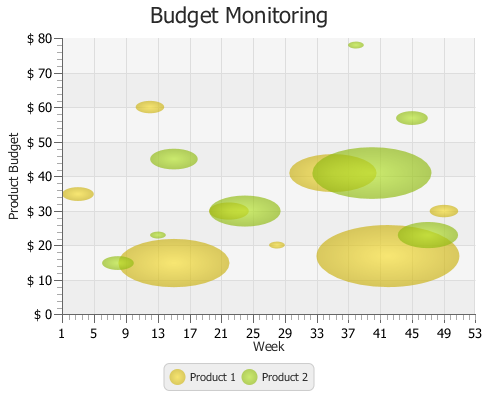Description of "Figure 5-4 Demonstrating Percentage of Consumed Budget"

## Changing the Appearance Visual Setting of the Plot and Tick Marks

You can alter the appearance of the chart plot and axes. Examine some methods and properties available in the JavaFX SDK API to modify the look of the Budget Monitoring application.

There are four properties in the `XYChart` class to manage the appearance of the chart plot. The `alternativeColumnFillVisible` and `alternativeRowFillVisible` properties define whether the alternative rows and columns have the fill colors. The `verticalGridLinesVisible` and `horizontalGridLinesVisible` properties enable and disable showing the grid lines. Table 5-1 shows the default values for these properties.

Table 5-1 Default Values of the Chart Plot Properties

Property Default Value

`alternativeColumnFillVisible`

`false`

`alternativeRowFillVisible`

`true`

`verticalGridLinesVisible`

`true`

`verticalZeroLineVisible`

`true`

Alter these properties by using the corresponding methods as shown in Example 5-4 to modify the look of the chart plot.

Example 5-4 Visualizing Alternative Columns

```blc.setAlternativeColumnFillVisible(true);
blc.setAlternativeRowFillVisible(false);
```

In addition to the plot properties, you can modify the properties of the axis to attain the required appearance. Code lines in Example 5-5 specify the `CHOCOLATE` color for the tick labels.

Example 5-5 Defining a Fill Color for the Tick Labels

```xAxis.setTickLabelFill(Color.CHOCOLATE);
yAxis.setTickLabelFill(Color.CHOCOLATE);
```

Finally, you can adjust the position of the tick labels relative to the tick marks. Use the `setTickLabelGap` method to specify the gap between tick labels and the tick mark lines on the vertical axis: `yAxis.setTickLabelGap(10)`.

Figure 5-5 shows the output of the Budget Monitoring application after all the modifications are incorporated in the code.

Figure 5-5 Changes in the Appearance of the Bubble Chart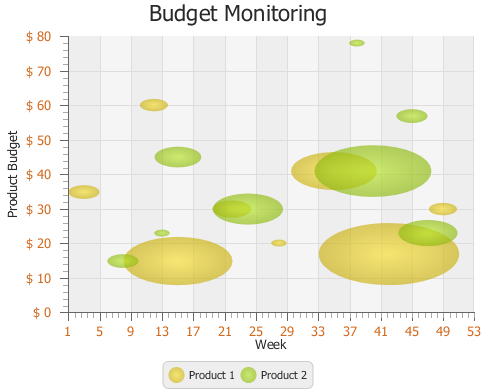Description of "Figure 5-5 Changes in the Appearance of the Bubble Chart"

Related API Documentation

• `BubbleChart`

• `Chart`

• `XYChart`

• `XYChart.Data`

• `XYChart.Series`

• `NumberAxis`

• `NumberAxis.DefaultFormatter`